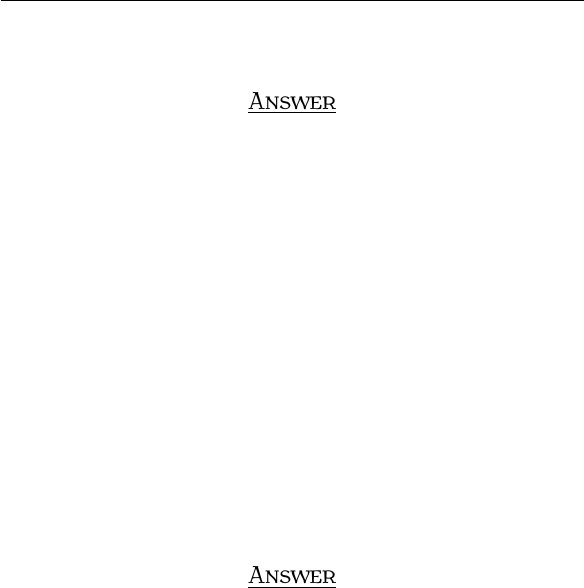Study Guides (400,000)
US (230,000)
BC (3,000)
MATH (90)
All (80)
Midterm

# Exam3210Sample5AnsExam

Department
Mathematics
Course Code
MATH 2211
Professor
All
Study Guide
Midterm

This preview shows pages 1-2. to view the full 6 pages of the document.MT T  S 
I S. Y
A , 
S, N:
Q . INTRODUCTION TO DETERMINANTS
Specify whether the matrix has an inverse without trying to compute the inverse
1 1 1 0 0
0 0 1 0 0
0 0 1 1 0
0 1 1 0 1
11 1 1 0
.
A
We use the deﬁnition of determinant. We calculate the determinant across the 2nd rows and 3rd column.
1 1 1 0 0
0 0 1 0 0
0011 0
0 1 1 0 1
11 1 1 0
=(1)
1 1 0 0
0 0 1 0
0 1 0 1
11 1 0
=(1)
(1)
1 1 0
0 1 1
11 0
=(1) ((1) ((1)
1 1
11
))
= (1)(1)(1)((1)(1) (1)(1))
= 0.
Since we have the determinant is 0, the matrix is NOT invertible.
1

Only pages 1-2 are available for preview. Some parts have been intentionally blurred.Q . THE PROPERTIES OF DETERMINANTS
(a) An n×nmatrix A is called skew-symmetric if At=A. Show that if Ais skew-symmetric and nis
an odd positive integer, then Ais not invertible.
A
By the properties of determinant,
det(At) = det(A)
det(A) = det(A)
det(A) = (1)ndet(A)
det(A) = det(A).
So, we get det(A) = 0 which implies that Ais not invertible. Note that Ameans that EVERY ROW of A
is multiplied by -1.
(b) Let A=
1λ0
111
001
. Determine those values of λfor which Ais invertible.
A
A=
1λ0
111
001
B=
1λ0
0 1 λ1
0 0 1
.
By IMT, Ais invertible if and only if det(A) = det(B)̸= 0. Thus, Ais invertible if and only if 1λ̸= 0 or
λ̸= 1.
2